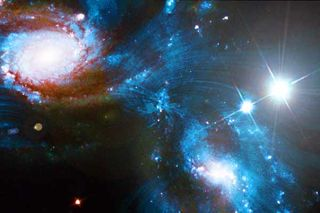# What's the Total Energy In the Universe?Considering the amount of energy packed in the nucleus of a single uranium atom, or the energy that has been continuously radiating from the sun for billions of years, or the fact that there are 10^80 particles in the observable universe, it seems that the total energy in the universe must be an inconceivably vast quantity. But it's not; it's probably zero.

Light, matter and antimatter are what physicists call "positive energy." And yes, there's a lot of it (though no one is sure quite how much). Most physicists think, however, that there is an equal amount of "negative energy" stored in the gravitational attraction that exists between all the positive-energy particles. The positive exactly balances the negative, so, ultimately, there is no energy in the universe at all.

Negative energy?

Stephen Hawking explains the concept of negative energy in his book The Theory of Everything (New Millennium 2002): "Two pieces of matter that are close to each other have less [positive] energy than the same two pieces a long way apart, because you have to expend energy to separate them against the gravitational force that is pulling them together," he wrote.

Since it takes positive energy to separate the two pieces of matter, gravity must be using negative energy to pull them together. Thus, "the gravitational field has negative energy. In the case of a universe that is approximately uniform in space, one can show that this negative gravitational energy exactly cancels the positive energy represented by the matter. So the total energy of the universe is zero."

Astrophysicists Alexei Filippenko at the University of California, Berkeley and Jay Pasachoff at Williams College explain gravity's negative energy by way of example in their essay, "A Universe From Nothing": "If you drop a ball from rest (defined to be a state of zero energy), it gains energy of motion (kinetic energy) as it falls. But this gain is exactly balanced by a larger negative gravitational energy as it comes closer to Earth’s center, so the sum of the two energies remains zero."

In other words, the ball's positive energy increases, but at the same time, negative energy is added to the Earth's gravitational field. What was a zero-energy ball at rest in space later becomes a zero-energy ball that is falling through space.

The universe as a whole can be compared to this ball. Initially, before the big bang, the universe-ball was at rest. Now, after the big bang, it is falling: light and matter exist, and they are moving. And yet, because of the negative energy built into the gravity field created by these particles, the total energy of the universe remains zero.

Ultimate free lunch

The question, then, is why the ball started falling in the first place. How did something – composed of equal positive and negative parts, mind you – come from nothing?

Physicists aren't exactly sure, but their best guess is that the extreme positive and negative quantities of energy randomly fluctuated into existence. "Quantum theory, and specifically Heisenberg’s uncertainty principle, provide a natural explanation for how that energy may have come out of nothing," wrote Filippenko and Pasachoff.

They continued, "Throughout the universe, particles and antiparticles spontaneously form and quickly annihilate each other without violating the law of energy conservation. These spontaneous births and deaths of so-called 'virtual particle' pairs are known as 'quantum fluctuations.' Indeed, laboratory experiments have proven that quantum fluctuations occur everywhere, all the time."

Cosmologists have constructed a theory called inflation that accounts for the way in which a small volume of space occupied by a virtual particle pair could have ballooned to become the vast universe we see today. Alan Guth, one of the main brains behind inflationary cosmology, thus described the universe as "the ultimate free lunch."

In a lecture, Caltech cosmologist Sean Carroll put it this way: "You can create a compact, self-contained universe without needing any energy at all."